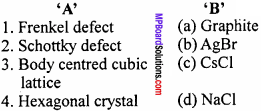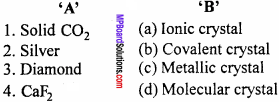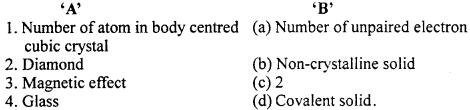# MP Board Class 12th Chemistry Important Questions Chapter 1 The Solid State

## MP Board Class 12th Chemistry Important Questions Chapter 1 The Solid State

### The Solid State Important Questions

The Solid State Objective Type Questions

Question 1.

Question 1.
Due to Frankel defect, density of ionic solids :
(a) Decreases
(b) Increases
(c) Does not change
(d) It changes.
(c) Does not change

Question 2.
In CsCl each Cl is surrounded by how many Cs :
(a) 8
(b) 6
(c) 4
(d) 2.
(a) 8

Question 3.
Frenkel defect is not shown by :
(a) AgBr
(b) AgCl
(c) KBr
(d) ZnS.
(c) KBr

Question 4.
In NaCl crystal number of oppositely charged ions situated at equal distance are:
(a) 8
(b) 6
(c) 4
(d) 2
(b) 6

Question 5.
Best conductor of electricity is :
(a) Diamond
(b) Graphite
(c) Silicon
(d) Carbon (non – crystalline).
(b) GraphiteQuestion 6.
Which type of point defect is found in NaCI crystal of KCl crystal: (MP 2009 Set D)
(a) Frenkel defect
(b) Schottky defect
(c) Lattice defect
(d) Impurity defect.
(b) Schottky defect

Question 7.
How many space lattices (Bravais lattice) can be obtained from various crystal systems :
(a) 7
(b) 14
(c) 32
(d) 230.
(b) 14

Question 8.
Diamond is a :
(a) H – bond solid
(b) Ionic solid
(c) Covalent solid
(d) Glass
(c) Covalent solid

Question 9.
The Co – ordination number of Ca2+ ions in fluoride structure is :
(a) 4
(b) 6
(c) 8
(d) 3.
(c) 8

Question 10.
8 : 8 Co – ordination number is found in which compound :
(a) MgO
(b) A1203
(C) CsCl
(d) All of these
(C) CsCl

Question 11.
Co – ordination number of body centred cubic cell is :
(a) 8
(b) 12
(c) 6
(d) 4
(a) 8

Question 12.
Density of unit cell is :
(a) $$\frac { ZM }{ { a }^{ 3 }{ N }_{ 0 } }$$
(b) $$\frac { Z{ N }_{ 0 } }{ { a }^{ 3 }M }$$
(c) $$\frac { { N }_{ 0\quad }{ a }^{ 3 } }{ Z }$$
(d) $$\frac { Z }{ M{ N }_{ 0 } }$$
(a) $$\frac { ZM }{ { a }^{ 3 }{ N }_{ 0 } }$$

Question 13.
The number of tetrahedral voids in unit cell of cubic close packing :
(a) 4
(b) 8
(c) 6
(d) 2
(b) 8

Question 14.
Intra – ionic distance of CsCl will be :
(a) a
(b) $$\frac {a}{2}$$
(c) $$\frac { \sqrt { 3 } a }{ 2 }$$
(d) $$\frac { 2a }{ \sqrt { 3 } }$$
(c) $$\frac { \sqrt { 3 } a }{ 2 }$$

Question 15.
Number of atoms in a body centred cubic unit cell is : (MP 2011)
(a) 1
(b) 2
(c) 3
(d) 4.
(b) 2

Question 16
Which of the following is Bragg equation :
(a) nλ = 2ϕ sinθ
(b) nλ = 2d sinθ
(c) nλ = sinθ
(d) n$$\frac {θ}{2}$$ = $$\frac {d}{2}$$ sinθ.
(b) nλ = 2d sinθ

Question 17.
Constituents of covalent crystal is :
(a) Atom
(b) Molecule
(c) Ion
(d) All of these.
(a) Atom

Question 18.
Number of Na atom present in the unit cell of NaCI crystal is : (MP 2012)
(a) 1
(b) 2
(c) 3
(d) 4.
(d) 4.

Question 19.
What type of magnetic substance are Fe, Co, Ni: (MP 2012,18)
(a) Paramagnetic
(b) Ferromagnetic
(c) Diamagnetic
(d) Antiferromagnetic.
(b) Ferromagnetic

Question 20.
The correct example of Frenkel defect is : (MP 2012)
(a) NaCI
(b) CsCl
(c) KCl
(d) AgCl.
(d) AgCl.Question 21.
Dry ice (solid CO2) is a/an : (MP 2012)
(a) Ionic crystal
(b) Covalent crystal
(c) Molecular crystal
(d) Metallic crystal.
(c) Molecular crystal

Question 22.
Co – ordination number of Cs in CsCl: (MP2015)
(a) Like Cl i.e., 8
(b) Unlike Cl i.e., 6
(c) Unlike Cl i.e., 8
(d) Like Cl i.e., 6.
(a) Like Cl i.e., 8

Question 23.
Structure of NaCI crystal: (MP 2015)
(a) Tetragonal
(b) Cubic
(c) Orthorhombic
(d) Monoclinic.
(b) Cubic

Question 24.
Each Na+ion in NaCI crystal is surrounded by :
(a) Three Cl ions
(b) Eight Cl ions
(c) Four Cl ions
(d) Six Cl ions.
(d) Six Cl ions.

Question 25.
For increasing of electro conductivity in a solid crystal, mixing of impurities is known as : (MP2016)
(a) Schottky defect
(b) Frenkel defect
(c) Doping
(d) Electronic defect.
(c) Doping

Question 26.
Which type of lattice is found in KCl crystal:
(a) Face centred cubic
(b) Body centred cubic
(c) Simple cubic
(d) Simple tetragonal.
(a) Face centred cubic

Question 27.
Number of atoms in a body centred cubic unit cell of a monoatomic substance is :
(a) 1
(b) 2
(c) 3
(d) 4
(b) 2Question 28.
Radius ratio limit for tetrahedral symmetry is :
(a) 0155
(b) 0.414
(c) 0.732
(d) 0.225
(d) 0.225

Question 29.
The defect produced due to a cation and an anion vacancy in a crystal lattice is known as :
(a) Schottky defect
(b) Frenkel defect
(c) Crystal defect
(d) Ionic defect
(a) Schottky defect

Question 30.
If co – ordination number of Cs+ is 8 in CsCl then co – ordination number of Cl ion is :
(a) 8
(b) 4
(c) 6
(d) 12
(a) 8

Question 2.
Answer in one word / sentence :

1. Give two examples of metallic crystal.
2. Give two examples of covalent crystal.
3. Give two examples of ionic crystal.
4. What is the co – ordination number of F+ ion in CaF1?
5. What is the value of co – ordination number of hexagonal close packing structure?
6. What is the type of structure of NaCl crystal?
7. Give an example of body centred cubic cell.
8. Give an example of a compound which has both Schottky and Frenkel type of defect.
9. What types of crystal is SiC? (MP 2011)
10. Write Bragg equation. (MP2017)
11. What is effect on the density of a substance or crystal due to Schottky defect?
12. Write the formula of radius ratio.
13. Give two examples of amorphous or non – crystalline solid.
14. F – centres give colour of crystal due to whose presence? (MP 2018)

1. Copper, Nickel
2. Diamond, Graphite
3. NaCl, NaNO3
4. 4
5. 12
6. Cubic
7. CsCl
8. AgBr
9. Covalent solid
10. nλ = 2d sinθ
11. Due to Schottky defect density of substance decreases
12. Radius ratio = $$\frac { radiusofcation\quad { r }^{ + } }{ radiusofanion\quad { r- } }$$
13. Glass, plastic
14. Due to presence of free electron.Question 3.
Fill in the blanks :

1. The defect produced due to removal of a cation and an anion from a crystal lattice is called …………………. (MP 2018)
2. If in a crystal lattice a cation leaves its lattice site and occupies a space in the interstitial site then the defect is called ………………….
3. The cause of electric conduction of NaCl in its molten state are its ………………….
4. Due to …………………. defect the density of crystal decreases
5. Total …………………. types of crystal system are there.
6.  …………………. proposed the concept of atom for the first time.
7. The ratio of the cation and anion present in a crystal is known as ………………….
8. The process of adding small amount of impurities in an element or compound is called …………………. (MP 2018)
9. Total 14 types of unit cells are there which are known as ………………….
10. In NaCl crystal structure, co – ordination number of both Na+and Cl ion is ………………….
11.  …………………. defect is found in ZnS and AgCl crystal.
12. Due to Schottky defect, density of crystal ………………….
13. In metallic solids, conductivity is due to the presence of ………………….
14. Point defects are found in …………………. crystals.
15. Substances which are attracted in magnetic field are called ………………….
16. For a unit cell, if r = $$\frac { a }{ \sqrt { 8 } }$$, then it will be …………………. type of unit cell.
17. Conductivity of semiconductor …………………. on increasing temperature.

1. Schottky defect
2. Frenkel defect
3. Free ions
4. Schottky
5. Seven
8. Doping
9. Bravais lattice
10. Six
11. Frenkel
12. Decreases
13. Free electron
14. Ionic
15. Paramagnetic substance
16. Fcc
17. Increases.

Question 4.
Match the following:
I. (MP2014)1. (b)
2. (d)
3. (c)
4. (a)

II.1. (c)
2. (d)
3. (a)
4. (b)

III.1. (d)
2. (c)
3. (b)
4. (a)

IV. (MP2017)